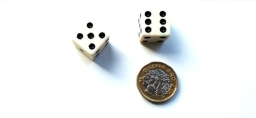# Probability 81842

In an opaque bag are chips with numbers from 1 to 20. What is the probability that we will draw a chip with a number less than 16?

p =  0.75

### Step-by-step explanation:Did you find an error or inaccuracy? Feel free to write us. Thank you!

Tips for related online calculators
Need help calculating sum, simplifying, or multiplying fractions? Try our fraction calculator.
Would you like to compute the count of combinations?